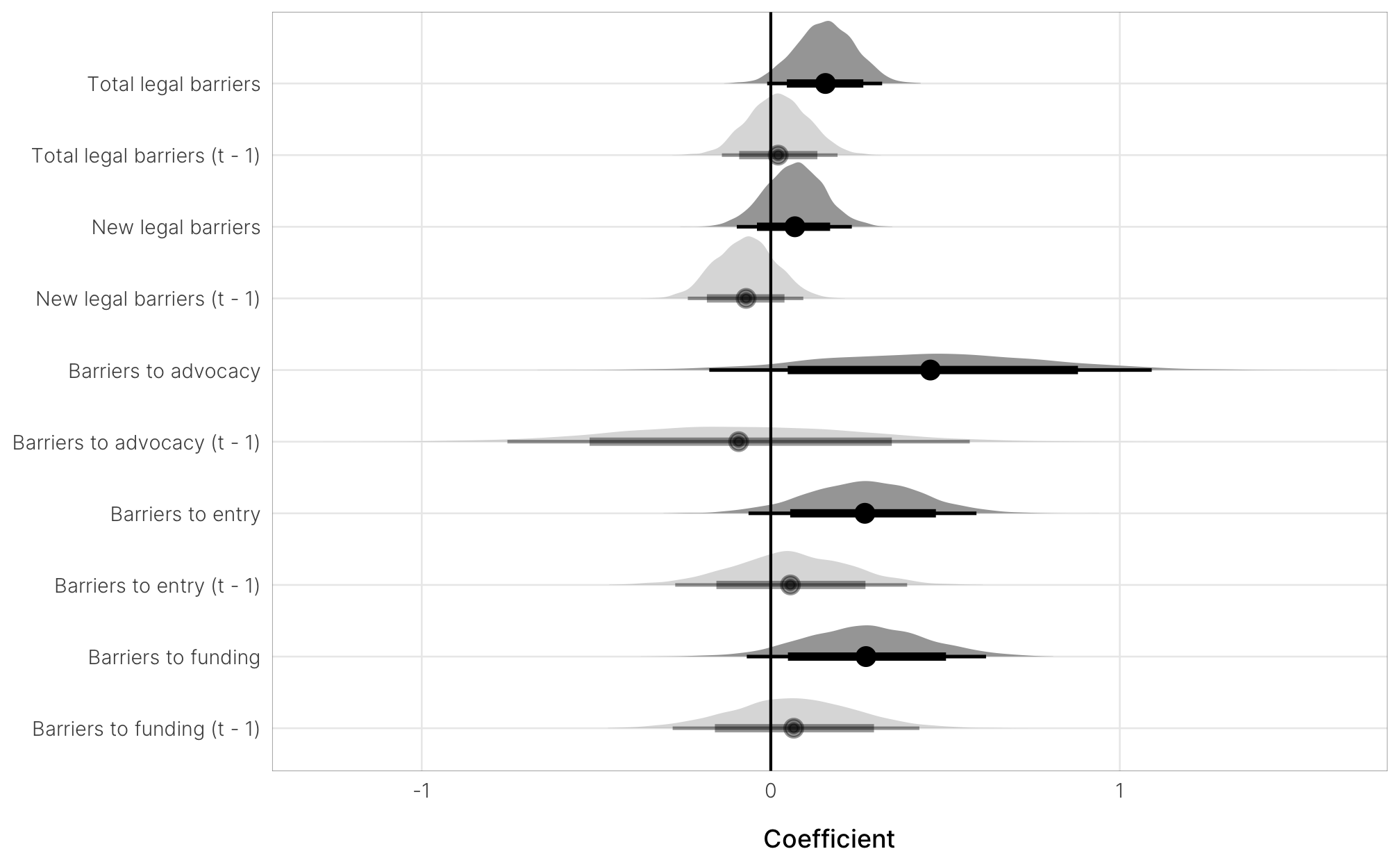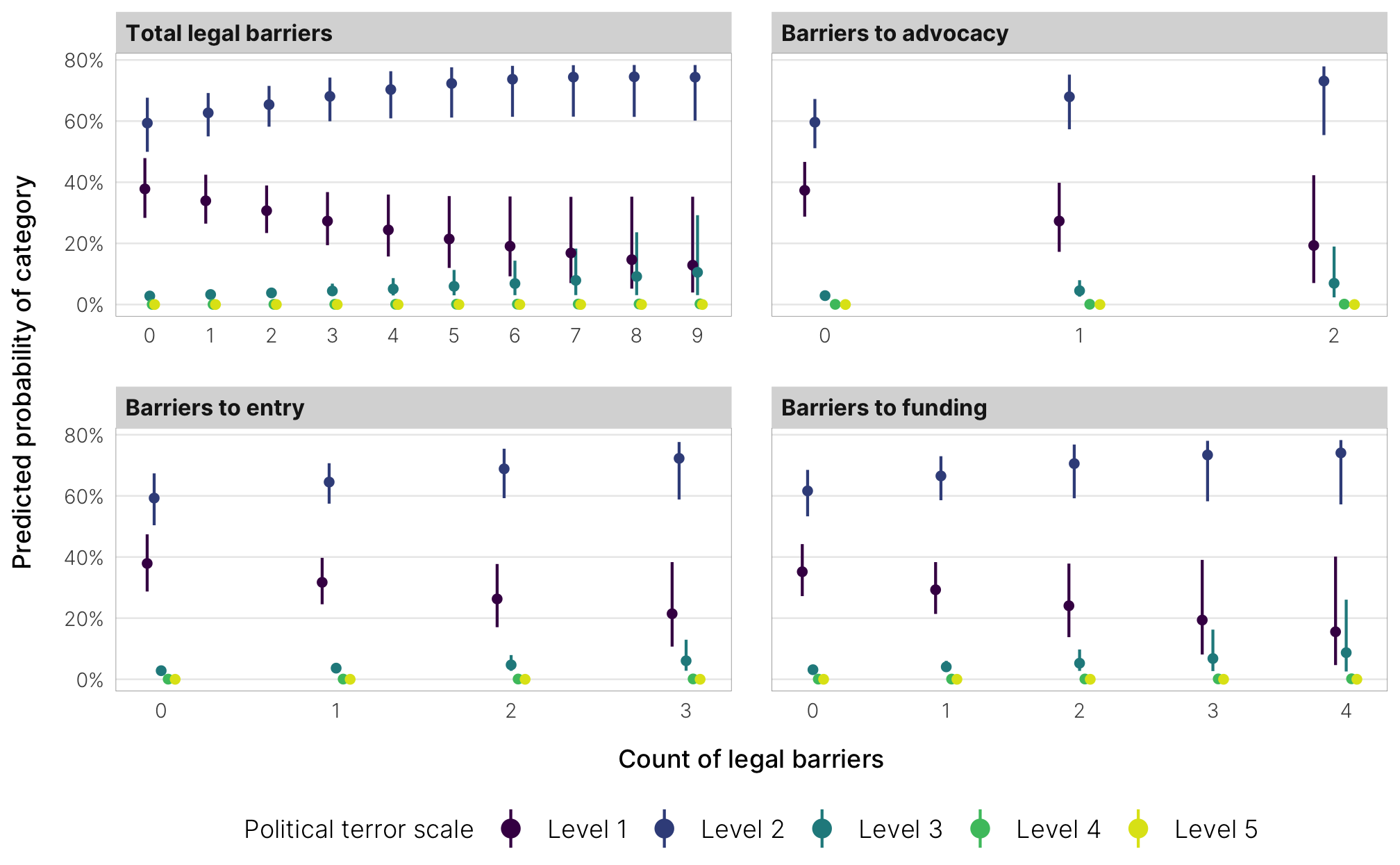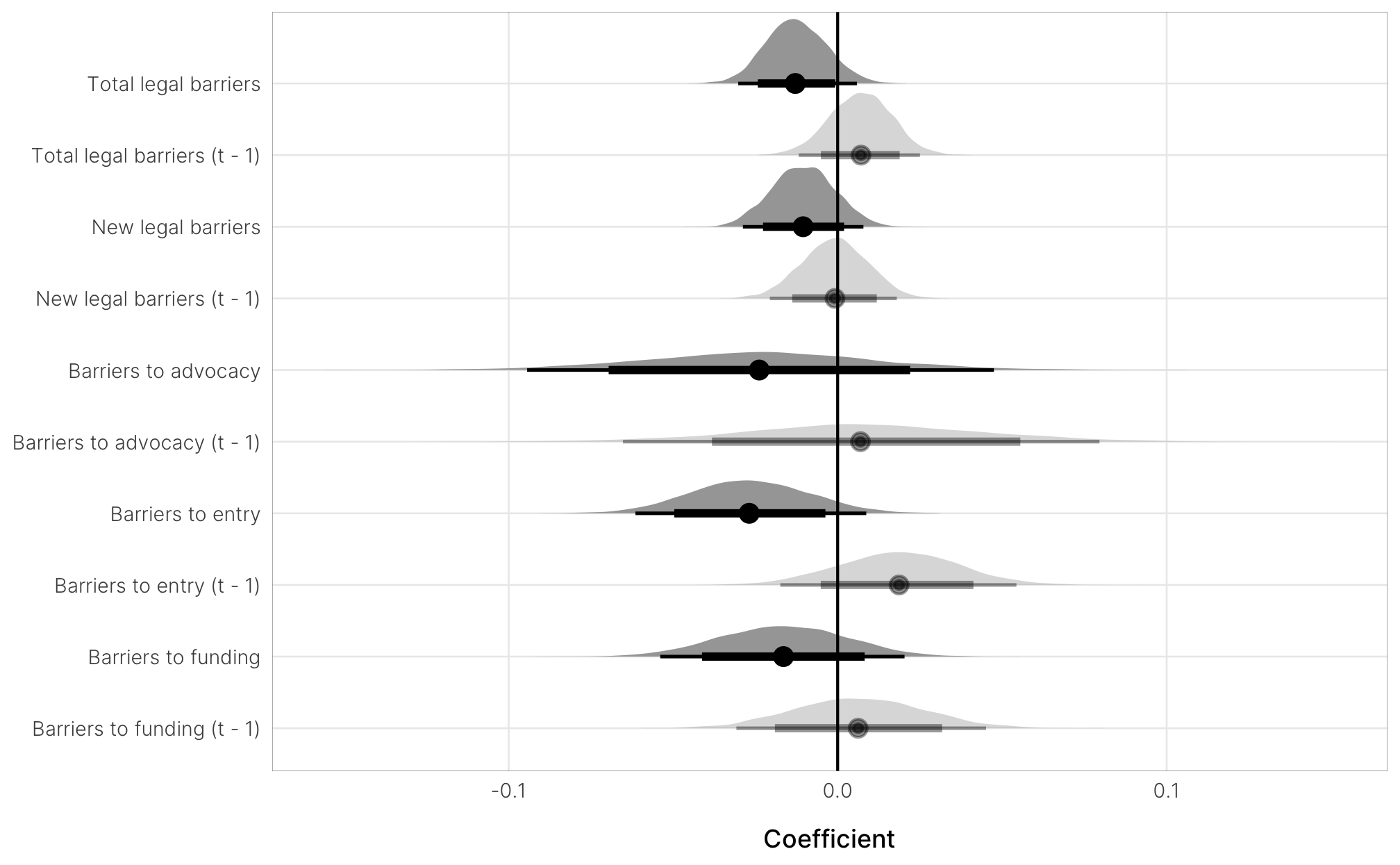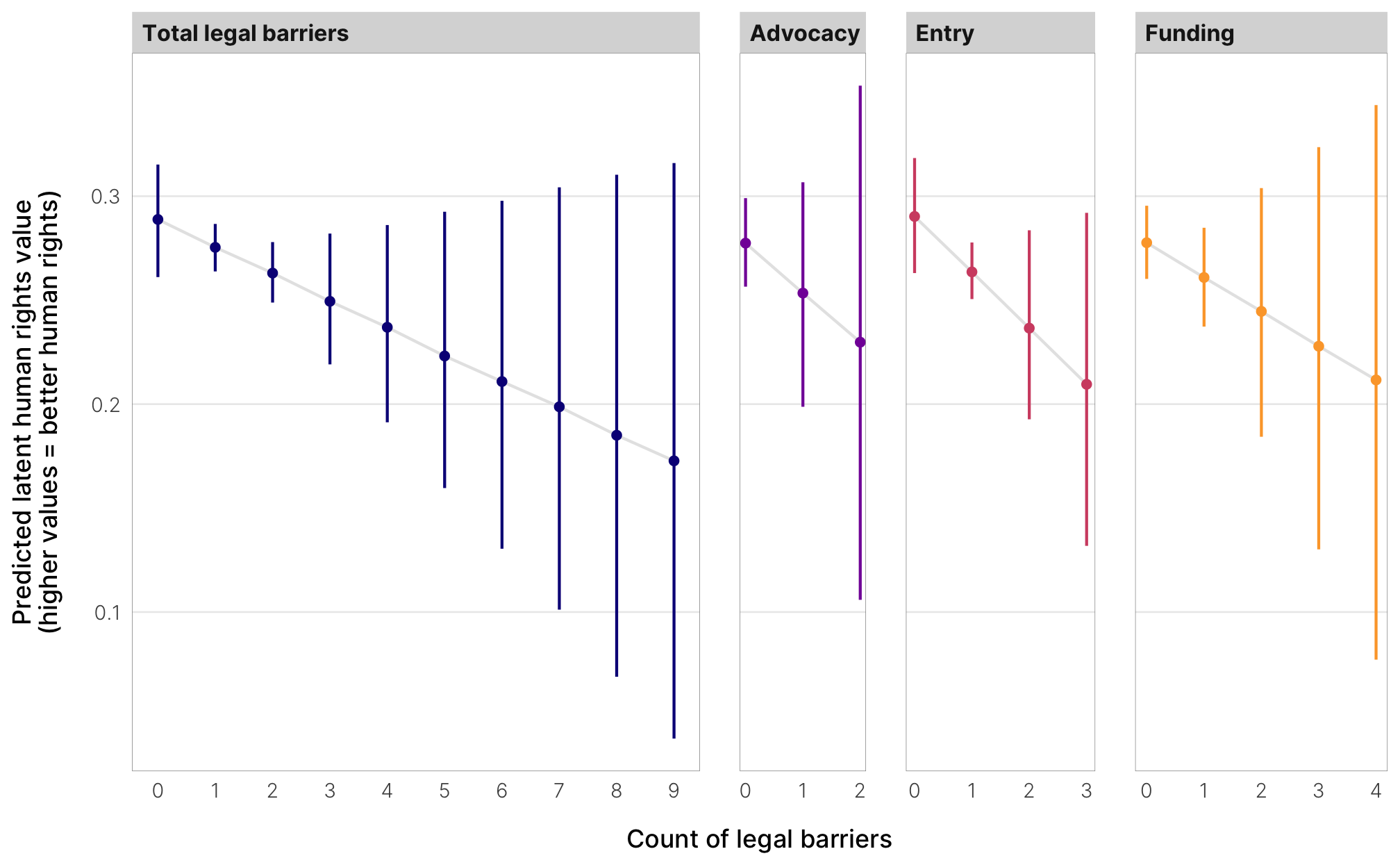library(tidyverse)
library(targets)
library(tidybayes)
library(glue)
library(brms)
library(scales)
library(kableExtra)
library(modelsummary)
library(here)

# Generated via random.org
set.seed(9936)

withr::with_dir(here::here(), {

# Load big list of models

m_lhr_baseline, m_lhr_total, m_lhr_total_new,

# Load lookup list for coefficients in regression tables
})
models <- model_df %>%
filter(!str_detect(model, "train")) %>%
mutate(actual_model = model %>% map(~eval(rlang::sym(.)))) %>%
mutate(across(c(outcome_var, explan_var, family), ~fct_inorder(., ordered = TRUE)))

coefs_clean <- tribble(
~coef, ~coef_clean,
"b_barriers_total", "Total legal barriers",
"b_barriers_total_lag1", "Total legal barriers (t - 1)",
"b_barriers_total_new", "New legal barriers",
"b_barriers_total_new_lag1", "New legal barriers (t - 1)",
"b_entry", "Barriers to entry",
"b_entry_lag1", "Barriers to entry (t - 1)",
"b_funding", "Barriers to funding",
"b_funding_lag1", "Barriers to funding (t - 1)",
"b_barriers_total_within", "Total legal barriers (within)",
"b_barriers_total_lag1_within", "Total legal barriers (within; t - 1)",
"b_entry_within", "Barriers to entry (within)",
"b_entry_lag1_within", "Barriers to entry (within; t - 1)",
"b_funding_within", "Barriers to funding (within)",
"b_funding_lag1_within", "Barriers to funding (within; t - 1)"
) %>%
mutate(across(everything(), ~fct_inorder(., ordered = TRUE))) %>%
mutate(lagging = str_detect(coef, "lag1"))

# Confounders

Time-varying:

• v2x_polyarchy
• GDP_log
• population_log
• armed_conflict

# E1a: NGO laws and political terror

## Results table

# Build nicer column names
models_for_table_e1a <- models %>%
filter(outcome_var == "Political terror") %>%
mutate(outcome_var = recode(outcome_var, "Political terror" = "PTS"),
explan_var = recode(explan_var, "Civil society repression" = "CS repression")) %>%
mutate(model_name = glue("{outcome_var} (t + 1)<br>({explan_var})"))

# Make a named vector of original model names and nice names
models_names_table_e1a <- models_for_table_e1a %>%
pull(model_name) %>%
set_names(models_for_table_e1a$model) # Rename the columns names(models_tbl_e1a) <- recode(names(models_tbl_e1a), !!!models_names_table_e1a) modelsummary(models_tbl_e1a, statistic = "[{conf.low}, {conf.high}]", coef_map = coef_list, gof_omit = "ELPD", escape = FALSE, notes = list("Posterior means; 95% credible intervals in brackets")) PTS (t + 1) (Baseline) PTS (t + 1) (Total legal barriers) PTS (t + 1) (New legal barriers) PTS (t + 1) (Barriers to advocacy) PTS (t + 1) (Barriers to entry) PTS (t + 1) (Barriers to funding) Total legal barriers 0.157 [−0.019, 0.308] Total legal barriers (t - 1) 0.021 [−0.144, 0.186] New legal barriers 0.069 [−0.101, 0.226] New legal barriers (t - 1) −0.071 [−0.233, 0.095] Barriers to advocacy 0.457 [−0.160, 1.100] Barriers to advocacy (t - 1) −0.092 [−0.730, 0.596] Barriers to entry 0.270 [−0.068, 0.580] Barriers to entry (t - 1) 0.056 [−0.256, 0.404] Barriers to funding 0.273 [−0.070, 0.616] Barriers to funding (t - 1) 0.066 [−0.284, 0.418] PTS = 2 2.399 2.250 2.213 2.288 2.259 2.257 [2.112, 2.688] [1.948, 2.542] [1.932, 2.539] [1.990, 2.583] [1.981, 2.572] [1.973, 2.580] PTS = 3 4.423 4.221 4.150 4.305 4.242 4.236 [4.057, 4.798] [3.857, 4.609] [3.786, 4.562] [3.926, 4.685] [3.867, 4.630] [3.868, 4.625] PTS = 4 6.518 6.227 6.223 6.344 6.255 6.215 [6.035, 6.978] [5.720, 6.696] [5.723, 6.713] [5.887, 6.878] [5.776, 6.733] [5.727, 6.692] PTS = 5 8.861 8.566 8.522 8.628 8.588 8.535 [8.272, 9.502] [7.924, 9.193] [7.841, 9.182] [8.012, 9.278] [7.977, 9.223] [7.906, 9.135] Polyarchy index −2.220 −2.215 −2.667 −2.302 −2.369 −2.239 [−2.889, −1.609] [−2.957, −1.555] [−3.367, −1.974] [−2.959, −1.610] [−3.095, −1.725] [−2.962, −1.589] Log GDP per capita −0.397 −0.471 −0.419 −0.434 −0.443 −0.471 [−0.539, −0.269] [−0.621, −0.320] [−0.573, −0.267] [−0.574, −0.288] [−0.584, −0.299] [−0.626, −0.321] Trade as % of GDP −0.368 −0.438 −0.380 −0.431 −0.422 −0.415 [−0.668, −0.068] [−0.751, −0.118] [−0.689, −0.017] [−0.743, −0.096] [−0.722, −0.097] [−0.729, −0.096] Armed conflict 1.010 1.083 1.050 1.067 1.077 1.089 [0.720, 1.326] [0.767, 1.411] [0.732, 1.369] [0.737, 1.363] [0.770, 1.375] [0.769, 1.392] Num.Obs. 3736 3594 3438 3594 3594 3594 R2 0.805 0.807 0.807 0.806 0.807 0.807 R2 Marg. 0.711 0.711 0.697 0.709 0.710 0.706 LOOIC 5456.1 5194.4 4960.8 5219.3 5199.7 5197.7 LOOIC s.e. 98.5 97.0 95.0 97.2 97.2 97.0 WAIC 5455.6 5193.8 4960.0 5218.6 5199.0 5197.0 Posterior means; 95% credible intervals in brackets ## Coefficients coef_plots <- models %>% filter(str_detect(model, "pts_"), !str_detect(model, "baseline")) %>% mutate(coef_draws = map( actual_model, ~gather_draws(model = ., .*barriers.*|.*advocacy.*|.*entry.*|.*funding.*, regex = TRUE))) %>% select(-actual_model) %>% unnest(coef_draws) %>% left_join(coefs_clean, by = c(".variable" = "coef")) coef_plots %>% ggplot(aes(y = fct_rev(coef_clean), x = .value)) + stat_halfeye(aes(alpha = lagging), .width = c(0.8, 0.95)) + geom_vline(xintercept = 0) + guides(fill = "none", alpha = "none") + scale_alpha_manual(values = c(1, 0.4)) + labs(x = "Coefficient", y = NULL) + theme_ngo()## Marginal effects plot_pts_vars_lookup <- tribble( ~model, ~plot_var, ~plot_var_nice, "m_pts_total", "barriers_total", "Total legal barriers", "m_pts_total_new", "barriers_total_new", "New legal barriers", "m_pts_advocacy", "advocacy", "Barriers to advocacy", "m_pts_entry", "entry", "Barriers to entry", "m_pts_funding", "funding", "Barriers to funding" ) %>% mutate(plot_var_nice = fct_inorder(plot_var_nice, ordered = TRUE)) mfx <- models %>% filter(str_detect(model, "_pts"), !str_detect(model, "baseline|new")) %>% left_join(plot_pts_vars_lookup, by = "model") %>% mutate(fx = map2(actual_model, plot_var, ~conditional_effects(.x, effects = .y, categorical = TRUE)[])) %>% select(-actual_model) %>% unnest(fx) mfx %>% mutate(x_rounded = floor(effect1__)) %>% group_by(x_rounded, effect2__, plot_var_nice) %>% slice(1) %>% ungroup() %>% ggplot(aes(x = x_rounded, y = estimate__, color = effect2__)) + geom_pointrange(aes(ymin = lower__, ymax = upper__), position = position_dodge(width = 0.2), fatten = 1) + scale_x_continuous(breaks = 0:9) + scale_y_continuous(labels = percent_format(accuracy = 1)) + scale_color_viridis_d(option = "viridis", end = 0.95) + labs(x = "Count of legal barriers\n", y = "Predicted probability of category", color = "Political terror scale", fill = "Political terror scale") + facet_wrap(vars(plot_var_nice), scales = "free_x") + theme_ngo() + theme(panel.grid.major.x = element_blank())# E1b: NGO laws and latent human rights ## Results table models_for_table_e1b <- models %>% filter(outcome_var == "Latent human rights") %>% mutate(model_name = glue("{outcome_var} (t + 1)<br>({explan_var})")) model_names_tbl_e1b <- models_for_table_e1b %>% pull(model_name) %>% set_names(models_for_table_e1b$model)

names(models_tbl_e1b) <- recode(names(models_tbl_e1b),
!!!model_names_tbl_e1b)

modelsummary(models_tbl_e1b,
statistic = "[{conf.low}, {conf.high}]",
coef_map = coef_list,
gof_omit = "ELPD",
escape = FALSE,
notes = list("Posterior means; 95% credible intervals in brackets"))
Latent human rights (t + 1)
(Baseline)
Latent human rights (t + 1)
(Total legal barriers)
Latent human rights (t + 1)
(New legal barriers)
Latent human rights (t + 1)
Latent human rights (t + 1)
(Barriers to entry)
Latent human rights (t + 1)
(Barriers to funding)
Total legal barriers −0.013
[−0.031, 0.005]
Total legal barriers (t - 1) 0.007
[−0.012, 0.025]
New legal barriers −0.011
[−0.029, 0.008]
New legal barriers (t - 1) −0.001
[−0.020, 0.018]
[−0.092, 0.049]
Barriers to advocacy (t - 1) 0.007
[−0.064, 0.080]
Barriers to entry −0.027
[−0.062, 0.008]
Barriers to entry (t - 1) 0.019
[−0.018, 0.053]
Barriers to funding −0.016
[−0.053, 0.021]
Barriers to funding (t - 1) 0.006
[−0.030, 0.046]
Latent human rights (t) 0.965 0.964 0.965 0.964 0.964 0.965
[0.954, 0.975] [0.951, 0.975] [0.953, 0.976] [0.952, 0.975] [0.951, 0.976] [0.952, 0.975]
Polyarchy index 0.090 0.074 0.096 0.080 0.082 0.076
[0.053, 0.130] [0.032, 0.115] [0.055, 0.141] [0.040, 0.126] [0.040, 0.126] [0.032, 0.117]
Log GDP per capita 0.006 0.008 0.006 0.007 0.007 0.008
[0.000, 0.014] [−0.001, 0.015] [−0.001, 0.014] [0.000, 0.015] [−0.001, 0.015] [0.000, 0.016]
Trade as % of GDP 0.022 0.024 0.023 0.024 0.024 0.023
[0.003, 0.041] [0.002, 0.044] [0.004, 0.045] [0.004, 0.044] [0.003, 0.044] [0.004, 0.043]
Armed conflict −0.007 −0.004 −0.002 −0.005 −0.004 −0.005
[−0.031, 0.019] [−0.029, 0.021] [−0.028, 0.024] [−0.032, 0.019] [−0.030, 0.022] [−0.030, 0.022]
Intercept −0.076 −0.073 −0.080 −0.075 −0.073 −0.076
[−0.135, −0.019] [−0.136, −0.010] [−0.144, −0.021] [−0.133, −0.011] [−0.138, −0.010] [−0.136, −0.014]
Num.Obs. 3769 3609 3449 3609 3609 3609
R2 0.968 0.969 0.968 0.968 0.969 0.968
R2 Marg. 0.968 0.968 0.967 0.968 0.968 0.968
LOOIC 118.9 47.9 135.9 49.4 51.5 49.3
LOOIC s.e. 352.5 351.7 342.5 351.1 352.4 351.2
WAIC 118.3 46.5 134.6 48.4 50.1 48.6
RMSE 0.79 0.79 0.79 0.79 0.79 0.79
Posterior means; 95% credible intervals in brackets

## Coefficients

coef_plots <- models %>%
filter(str_detect(model, "lhr"), !str_detect(model, "baseline")) %>%
mutate(coef_draws = map(
actual_model,
~gather_draws(model = ., .*barriers.*|.*advocacy.*|.*entry.*|.*funding.*, regex = TRUE))) %>%
select(-actual_model) %>%
unnest(coef_draws) %>%
left_join(coefs_clean, by = c(".variable" = "coef"))

coef_plots %>%
ggplot(aes(y = fct_rev(coef_clean), x = .value)) +
stat_halfeye(aes(alpha = lagging), .width = c(0.8, 0.95)) +
geom_vline(xintercept = 0) +
guides(fill = "none", alpha = "none") +
scale_alpha_manual(values = c(1, 0.4)) +
labs(x = "Coefficient", y = NULL) +
theme_ngo()## Marginal effects

plot_lhr_vars_lookup <- tribble(
~model, ~plot_var, ~plot_var_nice,
"m_lhr_total", "barriers_total", "Total legal barriers",
"m_lhr_total_new", "barriers_total_new", "New legal barriers",
"m_lhr_entry", "entry", "Entry",
"m_lhr_funding", "funding", "Funding"
) %>%
mutate(plot_var_nice = fct_inorder(plot_var_nice, ordered = TRUE))

mfx <- models %>%
filter(str_detect(model, "lhr"), !str_detect(model, "new|baseline")) %>%
left_join(plot_lhr_vars_lookup, by = "model") %>%
mutate(fx = map2(actual_model, plot_var,
~conditional_effects(.x, effects = .y)[])) %>%
select(-actual_model) %>%
unnest(fx)

mfx %>%
mutate(x_rounded = floor(effect1__)) %>%
group_by(x_rounded, plot_var_nice) %>%
slice(1) %>%
ungroup() %>%
ggplot(aes(x = x_rounded, y = estimate__, color = plot_var_nice)) +
geom_line(size = 0.5, color = "grey90") +
geom_pointrange(aes(ymin = lower__, ymax = upper__),
fatten = 1) +
scale_x_continuous(breaks = 0:9) +
scale_color_viridis_d(option = "plasma", end = 0.8) +
guides(color = "none") +
labs(x = "Count of legal barriers",
y = "Predicted latent human rights value\n(higher values = better human rights)") +
facet_grid(cols = vars(plot_var_nice), scales = "free_x", space = "free_x") +
theme_ngo() +
theme(panel.grid.major.x = element_blank())